## Saturday, April 12, 2014

### Propeller Static & Dynamic Thrust Calculation - Part 2 of 2 - How Did I Come Up With This Equation?

Subscribe by Email!
By Gabriel Staples Written: 12 April 2014
Last Updated: 1 June 2015
-added Figure #s - 1 June 2015
-minor units correction - 16 Apr. 2014
-minor addition to section describing prop helical twist - 29 Apr. 2014
-additions & corrections to the bold portions of the "Application & Conjecturing" section - 4 May 2014

Related Articles:

Since posting my initial "Propeller Static & Dynamic Thrust Calculation" post, I have had many questions about where this equation comes from, and several requests to explain more.  I have even had college students ask me about the equation.  Additionally, this is my most popular post at the moment and is getting over 700 views per month, with the bulk of those hits being from Google Search results about propeller thrust.  So, I think it's time I explain more about the background of the equation.  Here goes.

First off, here's the nomenclature I will use:

F = thrust (N)
m_dot = mass flow rate (kg/s)
Ve = exit velocity of the airflow through the propeller (sometimes called the induced velocity, inflow velocity, or velocity induced by the propeller) (m/s)
Vac = aircraft airspeed/velocity (not ground speed) (m/s)
Vpitch = propeller pitch speed (m/s)
ρ = air density (kg/m3)
A = area through propeller, normal to airflow (ie: area that the propeller sweeps) (m2)
P = pressure (Pa, N/m2, or kg/(m*s2))
SLUF = Steady, Level, Unaccelerated Flight

Here is some nomenclature *not* used explicitly in this article, but you should at least be aware of it:
ηprop = propeller efficiency (-)
ηmotor&prop = power system efficiency (of the motor, propeller, ESC, and wiring combined) (-)
L = lift (N, or kg*m/s2)
D = drag (N, or kg*m/s2)
W = weight (kg)
CL = lift coefficient (-)
CD = drag coefficient (-)
q = dynamic pressure (Pa, N/m2, or kg/(m*s2))
S  = aircraft planform area (m2); could also be used as propeller planform area when looking at a propeller as a wing

Background Equations
To get started, we must go all the way back to Newton.  Many people say that Newton's 2nd Law states that "force equals mass times acceleration:" F = ma.  This is not quite what his law really states.  Rather, this is only a very special case of Newton's 2nd Law.  Newton's 2nd Law really states that "force is equal to the time rate of change of momentum," or F = d(mv)/dt, where momentum is mass times velocity, or mv.  In many cases, mass is constant, so it can be removed from the derivative, and you get the following:Equation 1: Newton's 2nd Law, where mass is held constant.
I should probably bring up Newton's 3rd Law here, which states that "for every action, there is an equal and opposite reaction."  A propeller produces thrust by accelerating air molecules.  If we could measure the acceleration of each air molecule by the propeller, and also know the mass of each molecule being accelerated, then the thrust produced by each air molecule would be the mass of the molecule times the acceleration of the molecule.  The total thrust of the propeller would be the sum of all of the individual thrusts produced by all of the air molecules being accelerated at any given instant.  Remember that to get F = ma above, we had to assume that the mass of each molecule is constant.  What happens, however, if we look at the problem a bit differently and assume that the velocity of each molecule is constant instead?  This brings us to our second approach.

A second approach is to consider a mass flow rate, or m_dot (written as m with a dot over it), instead.  Any time you see a dot over a variable, that means it is the derivative of the variable with respect to (w.r.t.) time.  So, m_dot = dm/dt.  Its units are kg/sec.  If we take Newton's 2nd Law and hold the velocity of each molecule constant instead, we get the following:Equation 2: Newton's 2nd Law, where velocity is held constant.
Now, we are considering the air molecules to have a mass flow rate through a propeller, at a constant velocity.  This is a very common starting point for jet propulsion engineers and rocket scientists, as this equation is very common in both of those fields.  For a static aircraft, we know that all of the velocity of the air molecules passing through a propeller contributes to the thrust since the air molecules started out stationary, and were accelerated to this velocity.

Therefore, propeller thrust, F, for a stationary (static) aircraft is:Equation 3:  Theoretical static thrust

For a moving aircraft, however, only the velocity of the air which is due to the air having been accelerated by the propeller is what contributes to the thrust.  In other words, only the change of velocity is what matters:Equation 4:  Theoretical dynamic thrust
Note that based on this equation, as the aircraft velocity, Vac, increases, thrust decreases.  This is due to the fact that the propeller exit velocity (or induced velocity) is approximately constant, and therefore the result of (Ve – Vac) approaches zero as the aircraft top speed is reached.

Since m_dot is equal to the density of the air times the cross-sectional area through which the air is flowing, times the velocity of the air, we get:Equation 5: m_dot (left) & thrust w/m_dot plugged in (right)
where A is the cross-sectional area, or the rotor disc area covered by a spinning propeller.  A is therefore the area of a circle:Equation 6: Area of a circle
where r is the propeller radius, and d is the propeller diameter, in units of meters.

Substituting in A above, we get the following for the theoretical propeller dynamic thrust equation:Equation 7: Theoretical propeller dynamic thrust equation with area substituted in
Simplifying, by factoring the area term out we get:Equation 8: Theoretical propeller dynamic thrust calculation
Remember, Ve is the exit velocity of the air, or the induced velocity of the air by a propeller, through a propeller, and Vac is the aircraft velocity (or, more specifically and accurately stated, the freestream velocity).  F is thrust, ρ is air density, and d is the propeller diameter.

Ve is assumed to be approximately equal to the pitch speed of the propeller.  The pitch of an RC propeller is a unit, usually in inches, and represents the theoretical distance forward which a propeller would move, based on its pitch angle, if it were rotated exactly one revolution in a magical air which is more like Jell-O.  Pitch speed is solely dependent upon the propeller RPMs and pitch, and is as follows: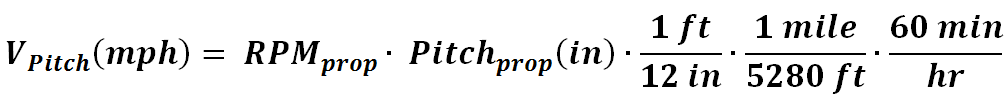Equation 9: Propeller pitch speed
Now, you can plug the pitch speed (Eqn. 9) into Eqn. 8, in place of Ve, to get an initial estimate for propeller thrust.  If you do this, however, you'll notice that the equation comes up with absolutely horrible estimates, and doesn't even show the appropriate trends for how thrust changes with varying diameter and pitch propellers.  Global trends are good when looking at changing diameter or pitch, independently, but if you look at changing diameter and pitch together, trends are not correct.  So, here comes the hard part, but first, let's briefly talk about the air density term.

To get air density, ρ, you could use the ideal gas law, P = ρRT.  Using this equation you can solve for density, rho, then plug in P, R, and T.  You can get P and T, respectively, by measuring static air pressure and temperature of the air where the propeller is being tested.  If you don't have an absolute pressure transducer, or a barometer, you can get static air pressure from a local weather station, using this equation:Equation 10:  Converting the local weather station pressure to the actual static pressure at that weather station’s altitude
where w.s. = “weather station.”
You can, of course, get temperature from a thermometer or from the local weather station too.  Make sure temperature is in Kelvin, and pressure in Pascals.  R is the specific gas constant of air, or 287 J/(kg*K).

This equation is essential since all weather stations provide only static pressures corrected for altitude, as opposed to true, raw static pressures.

To keep things simple, however, and primarily since I have no idea what the static pressures were during each of the thrust data points I collected from other sources, I just had to assume sea level standard day, so I assumed that air density, ρ = 1.225 kg/m3

Now, setting Vac to zero, we have the following for the static thrust calculation, with all pieces plugged in, and prop diameter, d, and prop pitch, pitch, being in units of inches, and thrust, F, coming out in units of newtons (N).  The 0.0254 is a conversion factor to convert inches to meters, since there are 0.0254m/in.Equation 11: Final theoretical static thrust calculation
At this point it is time to figure out empirical correction factors.

Theoretical to Empirical: Making the Equations Work For Real Life

At this point we are ready to find empirical, or based on experimental data, correction factors to make the thrust equation accurate.  Now that it is based on some physics, we have something to start with.  However, it is important to note that the thrust equation derived above has some huge assumptions.  For instance, the assumption that induced velocity is approximately equal to pitch speed (Ve ≈ Vpitch) is far from real life.  Not only is it unrealistic, but we also need to realize that the inflow velocity isn't even constant across the cross-sectional inflow area, or rotor disc area.

The helical twist on the propeller is an attempt to make the inflow velocity constant across the rotor disc area, but due to many factors, it is not.  Some of these factors include:
1) Non-constant inflow velocity profile across the propeller: I'd have to double-check to find the precise value, but to make my point: the majority of the thrust is produced somewhere around the 70% span, measured as 0.7 x r from the propeller hub, towards the propeller tip, where r is the prop. radius.  This is for some of the following reasons: the propeller is like a wing moving through the air, where the lift (thrust in this case) is proportional to the square of the linear velocity of the air striking the wing.  The linear velocity of the air striking the propeller is linearly proportional to the distance from the hub.  Therefore, maximum linear velocity occurs at the propeller tip, and at the center of the hub, it is zero.  To compensate for this velocity difference, the helical twist is such that the propeller near the tip will have a relatively low angle of attack to the air, but the propeller near the hub will have to have a much higher angle of attack to the air.  However, the motor gets in the way at the hub, no blade can exist at the hub, and even if blade could exist at the hub, the linear velocity at the hub is zero.  So, around the hub will be a low-flow zone.  Additionally, the angle of attack of the propeller blades near the hub may be high enough that the blade is stalled in that region.  A stalled blade can still produce thrust, but not nearly as much as an unstalled blade, and not nearly as efficiently.  Around the propeller tips, the inflow velocity will also suffer due to energy losses due to propeller tip vortices.  Therefore, the maximum inflow velocity, and consequently, thrust, occurs somewhere around the 70%~80% radial span of the prop, measured from hub to tip.
3) Propeller blade chord, planform area, and shape--I don't even take these things into account at all in my equation, as I wanted to keep the equation simple.

These are just two factors I can think of, but the point is that (Ve ≈ Vpitch) is truly a poor assumption.  Since it's the best I've got, however, I'll work with it.

At this point I started collecting static thrust data, from as many sources as I could find, to develop my empirical correction constants.  I needed to know at a bare minimum the propeller pitch, diameter, and RPMs, as well as the measured thrust for each data point.  I ended up finding 149 static thrust data points to use, from places like OS engines, Grayson Hobby, and even HobbyKing, pulling a few of the data points straight out of the user reviews.

Here are the 149 data points, for a whole variety of propeller sizes and RPMs.  The thrust values above 4kg were for large aircraft, some running gasoline engines and wooden props I think, but most from OSEngines, running electric motors on 6~7S LiPos.  The low line of values along the bottom right, going up past 22,000 RPMs, were the values from Grayson Hobby, as RCPowers (their affiliates), like to use very high-kV motors and small 5x5~6x4 props on their parkjets, which makes for very high RPMs but relatively low static thrusts.  The bulk of the data, in the "cloud" around 7,000~12,000 RPMs, is primarily from the OSEngines page previously linked above.Figure 1: Calculated vs. measured static thrust, for various props and RPMs

When I first plotted my estimates, compared to the actual thrust values, my estimates were *not* as you see them on the plot now.  Rather, they were far off of the real values.  To correct my equation I did the following:

First, I logically deduced (or rather hypothesized) that both the thrust, and also efficiency, of a prop must be related to its diameter to pitch ratio, since the diameter of a prop directly affects the incident (tangential) velocity (and hence also dynamic pressure and Reynold’s number) of air striking the blade at any given location of radial distance, r, from the hub.  Hence, larger diameter props will have not only increased thrust, but also increased efficiency over a prop of the same pitch but smaller diameter, since Re will be higher (and flow remain more attached) as you increase r.  However, pitch must also affect not only thrust but also efficiency.  For any two props of the same type and diameter, but different pitches, the prop with lower pitch will have lower incident angle of attack than the prop of higher pitch.  Lower incident angle of attack means better flow attachment, less separation, less induced drag, and therefore less profile drag/pressure drag too.  Therefore, higher diameter and lower pitch props must be more efficient, but how are diameter and pitch related?  Well, I decided to do a constant times the diameter to pitch ratio, then use “goal seek” in Excel on this constant, to find out what value for that constant gave me the lowest deviation of calculated static thrust from actual static thrust.  At this point, the equation looked like this, where I was goal-seeking to find the optimal value for the unknown empirical constant, k:Equation 12: First attempt at finding an empirical correction factor, k*(d/pitch), in this case, using goal-seek in Excel to optimize k

Pretty soon, however, I realized that the relationship of d/pitch to static thrust was nonlinear, so I added in a second constant to raise the diameter-to-pitch ratio to some power, as shown here:Equation 13: Second empirical attempt, this time with two unknown empirical constants, k1 and k2
where k1 is the "coefficient constant" I describe below, and k2 is the "power constant."
I then used trial and error to juggle around the two constants for several hours, incrementally changing one, and goal-seeking the other.  Repeated trials led me to choose 1.5 as the value for the power constant, k2.  This value is still enigmatic to me, and I do now know why it works best.  The value 1.5, or 3/2, just seems to be too perfect, and therefore perhaps has an analytical basis, but I just don’t know what.  After choosing 1.5 as the power constant, performing the goal-seek on k1, the coefficient constant for the diameter to pitch ratio, gave me a value of 1/3.29546 (or 0.30344777), as the optimal coefficient constant necessary to minimize the average error between the predicted and actual thrust values for the 149 data points I looked at.

At this point I was complete, and that brings me to my final static thrust equation at this time, shown here: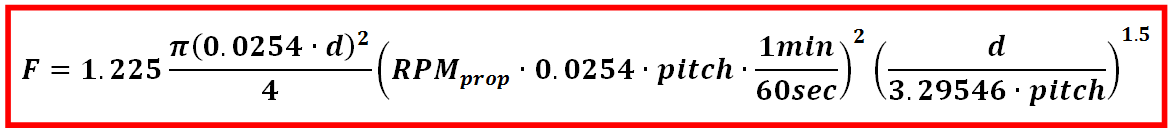Equation 14: Final static thrust equation, with empirical correction factor

Finally, I added Vac back in to the equation (refer back to Equation 8) in order to make it applicable to dynamic thrust calculations, and I compared it against one single dynamic thrust test run from Matthew McCrink of Ohio State University.  Since my equation produced reasonable results, even if only only for a single dynamic thrust data set, I consider it to be a success, though future work will include applying an empirical correction constant to the dynamic thrust portion of the equation as well, in order to improve the accuracy of the zero-thrust, or x-intercept point.  My static thrust empirical correction factor makes the y-intercept point accurate, so now if I can make the x-intercept point more accurate, I can simply draw a line between the two in order to have the complete dynamic thrust estimate for any given airspeed, Vac.  After that, I can worry about the minor non-linearity in the line segment that is noticeable between those two points, but it is so minor that perhaps it can just be ignored.  Additionally, I plan to look at some blade-element-theory type techniques, breaking the propeller up into small segments and independently looking at each segment, considering such things, perhaps, as apparent angle of attack to the inflow (to see which segments of the prop may be stalled at any given time), and inflow velocity for that segment of the propeller, since as discussed previously, I know the inflow velocity is not constant across the inflow area.  This will require using an annular (ring-like) cross-sectional inflow area (rather than circular), for each prop segment I look at, since I will be looking at only segments of the prop. at a time.  These are just some ideas that I have, however, as I'll have to see what's truly feasible as I think through and attempt the next steps.

One other idea, which I think is very feasible and will work very well, is to use multi-rotors/multicopters (ie: quadrotros, tricopters, hexacopters, etc) to further refine my static and dynamic thrust estimates.  For any vehicle in a steady, hovering state, the thrust is equal to the vehicle weight.  Knowing this, you can take a multirotor vehicle, hover it, use an Arduino microcontroller to do real-time measuring and datalogging of each motor's RPMs (I've already worked out an initial version of this code), and then apply my equation to refine the thrust estimate.  The thrust each propeller is producing is simply the total vehicle weight divided by the number of propellers on the vehicle.  Ex: for a standard quadrotor configuration, for a quadrotor weighing 1kg, the thrust of each propeller, in any given steady, horizontally-stationary (horizontal velocity = 0), unaccelerated hovering state, is 1kg/4.

Finally, here is my dynamic thrust equation, in its current final form, with the empirical correction constants, as discussed above, applied.  Note here, however, I previously chose to use V0 to represent the aircraft velocity, instead of Vac.  They are the same thing though, so ignore that minor difference.  These are the same equations that I show in Part 1 of this article.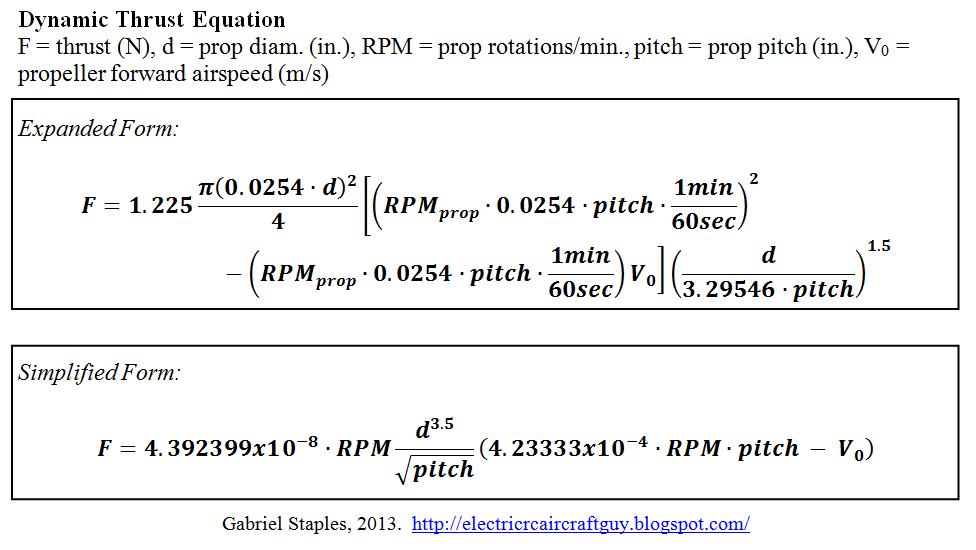Equations 15 (top) and 16 (bottom): Final, empirically-corrected, dynamic thrust equations for a propeller (top: expanded form, bottom: simplified form)

Here is the single dynamic thrust test point from Matthew McCrink.  I discuss this plot more thoroughly in Part 1 of this article.Figure 2: Propeller thrust: experimental results in wind tunnel vs. my semi-empirically calculated results from Eqn 15 or 16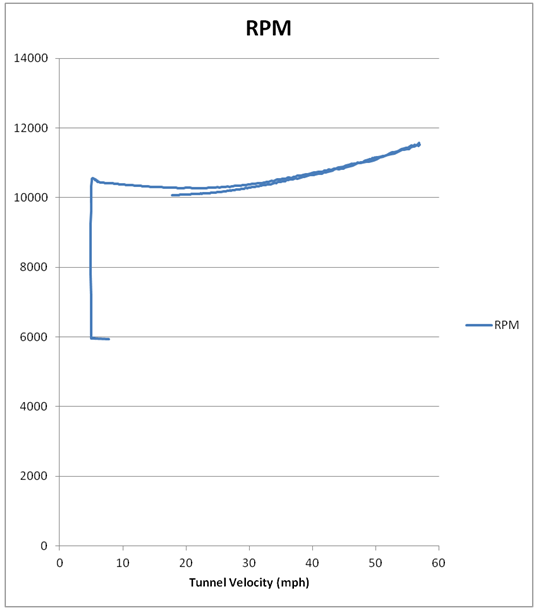Figure 3: Motor RPM vs wind tunnel velocity during test shown in Figure 2

Here is a diagram I am adding for completeness, in case you'd like to study it for additional insight: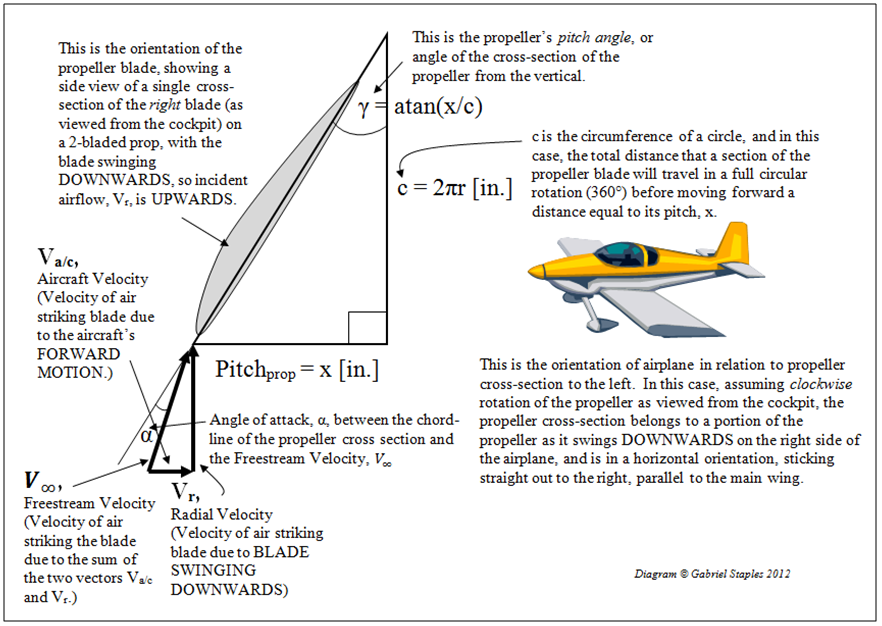Figure 4: Dynamic propeller physics, including propeller angle of attack during forward flight

Application & Conjecturing:

One potential application of my dynamic thrust equation is to look at how power system choice affects max speed of your aircraft.  Realistically, we would need some more info. about your aircraft, such as drag coefficient, so that in SLUF (Steady, Level, Unaccelerated Flight) we could assume thrust = drag, but let's just make some generalized statements to get my point across for now.  The point is that your airplane can never fly faster than the airspeed indicated at the x-intercept of the thrust vs. airspeed curve.  If your power system produces zero thrust at a given airspeed, then your plane will never fly quite that fast.  If it was perfectly drag-free, it would fly exactly that fast.  Therefore, my equation at least gives us a decent idea of what the max theoretical, drag-free airspeed might be for a given setup.  Using my equation, let's see how prop pitch, diameter, RPMs, and airspeed are related.  Let's take a look.

Using my dynamic thrust equation, I created this plot below, trying to use reasonable values I guestimated based on my personal RC experience, for one particular power system you might have.Figure 5: Conjectured thrust vs aircraft airspeed curves, showing the tradespace between prop selection

Notice that dynamic thrust is linear.  Based on my equation, this makes sense, because although thrust has a squared relationship to induced velocity through the propeller, it has only a linear relationship to aircraft speed, or airspeed.  Looking at Figure 4 on this website (http://www.allstar.fiu.edu/aero/BA-Form&gra.htm), you can see that thrust of a prop-driven airplane does indeed taper off almost linearly.  You can also see that in the wind tunnel data I posted above.

This is in contrast to jet airplane.  From a few plots I saw in my core aero book (ISBN-13: 978-1563477010, found here), for a jet airplane, thrust vs. airspeed is basically just a straight horizontal line.  I believe this is because for a jet airplane, V0 (ie: Vac) is insignificant compared to V(ie: Ve >> Vac), so thrust stays nearly constant across various aircraft airspeeds.

To make a point, looking at the various curves on the thrust vs. airspeed plot above, you can see that despite having the lowest static thrust, the smallest diameter, highest-pitch prop in this case (7x6 at 13,500 RPM) has the highest potential to get the plane going fast.  In other words, it has the highest high-speed dynamic thrust, since its curve intersects the x-axis the farthest to the right.  Assuming that your propeller/battery comparisons maintain constant power for a given motor (since a power system: motor, ESC, battery, etc., is in fact power limited, due to heat generation), that is always the tradeoff: high static thrust versus high dynamic thrust (& hence also high pitch speed, & high top speed).  In this made up case, the 7x6 prop. has the lowest static thrust, but the highest dynamic thrust.  Therefore, it might be a poor choice on this power setup for a 3D plane, which needs very high static thrust to be able to hover and accelerate vertically, but it might be ideal on a sport plane you want to fly around fast, since high-speed flight requires a high pitch speed and higher dynamic thrust.  The fast plane sacrifices high static thrust, as indicated by a lower thrust curve near the left of the plot, in exchange for higher dynamic thrust and top speed, as indicated by extending the curve to the right on the plot.   This trade-off in choosing your propeller is like shifting gears in a car.  Either you get a really good top speed with lousy initial acceleration (like 5th gear), or you get a really low top speed with really good initial acceleration (like 1st gear).  Assuming constant power draw through your given motor/battery/ESC setup, a 10x4.7 prop is more like 1st gear, and a 7x6 prop is more like 5th gear.

One more note: the area under each curve is equal to the Power of the propulsion system.  If put in standard metric units, the y-axis, thrust, would be in Newtons, and the x-axis, airspeed, would be in m/s.  The area under the curve would be in units of thrust x airspeed = Nm/s = J/s = W = Watts.  This is the unit of power.  There are 745.7 watts per horsepower (W/hp).

In order to make the hypothetical propeller comparisons above more realistic, I need to redo that plot, this time ensuring that the areas under the curves are equal, thereby ensuring constant power for each propeller being used.
[Todo: redo the above plot, ensuring equal areas under the curves, & thereby equal power draw for each setup (W = J/s = Nm/s = area under the curve).  Also, calculate the power for a given system, & see if this correlates accurately to the real-life measured power draw through a system, or if I am misunderstanding something ~GS.]

One more thing to keep in mind:  These plots above assume constant RPM.  However, as Vac increases, the RPM data from the wind tunnel experiment above shows that RPM also increases.  This seems explainable to me by the fact that effective Angle of Attack (AoA, or alpha) on the prop blades decreases with increasing airspeed (see my figure above, showing the side profile of the propeller).  Since effective AoA decreases, pressure drag and induced drag will decrease on the blades.  This, perhaps, is what allows the propeller RPM to increase.  I hypothesize that decreasing effective AoA on the prop blades reduces resistive torque, which allows the motor to speed up a little.  I will have to look into this phenomenon more in the future.  If you look at the Cessna 172 data in Figure 4 of this source, you'll see that the thrust curve is very slightly parabolic, bowing up in the center.  The wind tunnel data also slightly shows this phenomenon.  I would like to know what causes this, so that is something else I will look into in the future, as I continue learning and growing.

That's it for now!

If you have not read my precursor article, "Propeller Static & Dynamic Thrust Calculation, Part 1 of 2", I highly recommend you do that.

***Subscribe by Email!***

1.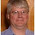1.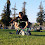Sorry, I have no data nor equation for 3 or 4-bladed props yet. Please see my lengthy response, below Part 1 of this article, to a comment by "Thinqer", from September 24, 2013 at 9:34 AM.

2.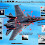Hi,

did you mean V0 was equal to the cruise speed of the propeller while moving forward in the air?

3.V0 = propeller forward air speed = Vac = aircraft airspeed for a fixed-wing aircraft = propeller inflow velocity. They are all the same thing.

Cruise speed is something else. Cruise speed is a typical airspeed for a given airframe, which is determined to be a relatively fast, economical, and "comfortable" airspeed for the given airframe. See here: http://www.oxforddictionaries.com/us/definition/american_english/cruising-speed

1.ok noted that, thank you very much.

4.thank you very much, a very useful article, i may use the equation in my senior design project.

5.hi
i am designing a hexacopter, i'm using a dji E300 2212/920KV motor with 3s lipo battery that provides 11.1 v that means 10212 rpm, and lets assume a minimum thrust of 300g per motor, and propellers dimensions are 9.4X4.3 inches.

and if i used your final equation with Vac equal to zero it gives me about 10N
how's that possible?

1.10212 is the theoretical RPM for an *unloaded* motor, at that voltage. The moment you add a propeller, the RPM drops *dramatically*. You'd have to use the real RPM from experimental testing, or from a good online calculator or other equation, to determine the real RPM for this setup. You cannot use the theoretical *unloaded* RPM based on kv and voltage alone.

6.so first of all i have to determine the RPM when the propeller is loaded to the motor then use that number in your equation is that right ?

and two more things, the first one can i determine Vac theoretically?
the second one is Vac=0 when the drone is suspended in the air ?

7.i know that the point of your equation is to get the prop RPM after measuring the thrust experimentally, but the problem is that i am designing a drone on solidworks and matlab which they both deal with equations, i am not doing any kind of experiments.

any ideas ??

8.i think i understood everything thank you very much a very brilliant equation

9.Can you provide me with the units of pitch,diameter and rpm

1.Pitch has units of inches per revolution, diameter has units of inches, and RPM is a unit in and of itself: rotations per minute. If a propeller is listed as a 9x5 prop, for instance, 9 is the diameter in inches, and 5 is the pitch in inches forward movement per revolution. RPM is the rotational speed of the propeller in revolutions per minute. This can be obtained by testing the whole system, for example, and measuring the propeller RPM value with an optical tachometer, stroboscope, or electrical tachometer/oscilloscope reading the back EMF voltage readings across any one of the 3 motor windings and battery ground.

2.Please help me to find out motor propeller combination for my 21kg plane which will running on 6s lipo pack and has to power less then 1000watt.
24g.vijay@gmail.com

3.Vijay, this sounds like a university competition to carry as much weight as possible with <= 1kW power, and I'm guessing 21kg is the minimum required for an "A"? I'm sorry, I don't have the time to solve this problem for you. I do recommend, however, you use a good online sizing calculator like this one here. Also, check out this video from FliteTest. I don't know what their power draw was but they carried 21.8kg for < \$100 of parts. (Flite Test - Flying Cinder Block - PROJECT) Now that's impressive! Keep in mind that you'll get better efficiency with high-voltage batteries (like 6S or higher), low kV motors, low RPMs, and large-diameter propellers. If you require more diameter but don't have the ground clearance, add more blades (at the sacrifice of efficiency)--ex: 3 instead of 2 blades. Just make sure that the power system produces enough *velocity* in the thrust that you can get the plane above stall speed. If you take what I said too far, for instance, you'll end up with a large propeller that produces tons of thrust when not moving and no thrust at velocity--meaning it will never get the plane above stall speed even though the static thrust is fantastic. This is because as you increase propeller diameter towards infinity static thrust will also approach infinity but induced air velocity will approach zero. Hmmm. Interesting...Best of luck!

4.Clarification: when I say "tons of thrust when not moving" I mean "tons of thrust when the *plane* is not moving"--ie: tons of "static thrust."

Also, I forgot to mention: I used that online sizing calculator I mentioned above on my BattleBot flame-throwing hexacopter, "Buzz the fire drone", so I know it works. Plan to spend a good amount of time sizing things though. It's not easy.

10.Can we calculate for Thrust from a given value Torque?

11.It seems that some of the methodologies for choosing a power train for a given model start by estimating required and output engine wattage (assuming an engine efficiency) and maximum velocity, then use huge databases to choose a propeller. Is it practicable to develop a similar equation to your thrust equation to determine propeller input watts from diameter, pitch and RPM?

12.hi iam trying to build a big rov, and i cant find any reason to not use the equations you have deduced in this site (ill use water density instead of air).i want use this to find the right combination of thrust, propeller dimensions, rpm to decide right motors, propeller for the job.please let me know anything else should i change in your equation other than density. btw i am super impressed and fan of your site from now for the effort u have put in this, thanks on behalf of every hobbyist.

1.Hi, I can't think of anything to change other than density, but I really don't know how well the equation will work for water. Good luck and I'm glad you have found my site useful. Thanks for the gratitude!

13.I'm looking for a way to convert from Thrust to Electric Power.
I understand it'll need additional variables I believe np(Prop Efficiency) and nelec (electric Efficiency)
are required but I can't seem to figure out how to do this conversion. :-(

Do you have any recommendations or have you done this equation in the past?

14.Very nice study, thanks a lot for your work.

Regarding the expression for dynamic thrust, it is slightly different to the one derived at https://www.grc.nasa.gov/www/k-12/airplane/propth.html using Bernoulli's equation. In particular, this equation has a (ve^2-vac^2) dependence rather than being linear with the aircraft speed. Looking at your plot of experimental data for dynamic thrust, the points look close to linear but slightly convex, which will be what one would expect from the Bernoulli's derivation. So it looks like something in between. Any thoughts about that?

1.Great question. Thanks for sharing NASA's thrust derivation based on Bernoulli's equation. Whether you use Newton's equation like I did, or Bernoulli's equation, I've heard/probably even read somewhere in the past they are both equally valid, and if the same assumptions are used, should yield the same result. It's possible I've made some errors along the way, or different assumptions, but if you look at My Equation 5 it's actually surprisingly similar. I have V^2 - V^2 terms too. My Equation 5 says F = rho*A(Ve^2 - Ve*Vac), whereas the NASA equation has F = 0.5*rho*A(Ve^2 - Vac^2). Ve*Vac is very similar to Vac^2, but still different. Also, I go on to empirically correct my equation, whereas NASA simply left it as-is. Also, I don't require you have any idea what Ve actually is. Since it's very difficult to measure while flying, I just worked it right out of the equation so you could use the propeller constants and empirical correction constants instead of actually having to measure and know Ve. NASA doesn't take it that next step, so they expect you to know Ve, which isn't practical for most cases.

I'll see if I can contact NASA and find the author of that page they have. Maybe he and I can talk about it.

15.This comment has been removed by the author.

1.I'm guessing you removed your question because you figured it out. At the end of the Expanded form of Equation 15, you have (d/(3.295*pitch)^1.5. No unit conversion from inches to meters is necessary for d or pitch because they both have the same units, so units cancel.

16.can i use this equation to calculate propeller thrust of a quardcopter propellor

1.17.Hello,
How can we calculate the F without knowing the propeller efficiency...?
different brand props will have different geometry, aerofoil etc...

1.I don't account for any of that--which I'm pretty sure I even mention in one of these 2 related articles. This is a crude and generalized equation and considers somewhat of an empirical average of these characteristics, based on real experimental results of a variety of propellers.

18.Hi Gabriel, will this eqn work for an X8 octicopter configuration?

1.Sort of: I have not accounted for the inefficiencies related to stacked propeller configurations, but perhaps if you considered a hovering X8 octocopter to have its top propellers in a static thrust condition (no forced inflow velocity) and the bottom propellers to be in a dynamic thrust condition (with inflow velocity induced by the top propellers) you could make the equations work for this case.

19.Could you explain in more depth why increasing the propeller diameter, increases the reynolds number and efficiency?

20.what is the reference book of this equation?

1.No reference book. It's my equation, hence the title of this article: "How Did I Come Up With This Equation?" That title is intentional.

21.Hi Gabriel,

Thanks so much for your work on producing this equation! It is a great piece of work, and it's very valuable for a class that I'm teaching. In your explanation, you found that your equation had the best fit to empirical data when the final term (d/3.296*pitch) was raised to a power of k2=1.5 (or 3/2), which you found to be an enigmatic result. I think I discovered why the value of 1.5 was the best value for k2.

To understand this, let's break down your equation. The first term of your equation is expressed as: RPM * d^3.5 / pitch ^0.5. When you analyze the units associated with each variable, you get: Rev/min * in^3.5 / (in)^0.5. This can reduce to in^3/min, which is essentially a measure of the volume of air that is moving through the propellers per unit of time. When you multiply this value by the density of air (kg/m^3; after converting inches into meters), you can determine the total mass (in kg) moving through the propellers per unit of time.

When you analyze the units from the second term of your equation, you get m/s (i.e., a velocity).

When you multiply these two units together (after converting minutes into seconds), you get the following unit: kg*m/s^2, which you will recognize as an extremely important unit in physics (i.e., Newtons). Therefore, the only value of k2 that would yield a valid unit for Thrust (i.e, Newtons) is 1.5. Any other number would have yielded an invalid unit for this force.

Please let me know if you agree and whether I'm missing something.

John

1.Yes that was really very clever approach😇👍

22.This equation is applicable for multi rotors?

1.Yes, so long as the propellers are in a side-by-side configuration, NOT a stacked configuration. Ex: X-4 configuration is fine; just sum the 4 motor thrusts. X-8 configuration will have additional error though, because each arm has 2 motors with the propellers stacked on top of each other, causing thrust interference and meaning my equation is not as accurate for that situation. See also my comment here from September 29, 2021 at 10:49 AM.

23.when i get 0 thrust at a speed, does this means this is my maximum attainable speed of aircraft.

1.No I think it will be lower than that. It'll occur when thrust is equal to drag. For quadcopters it will be dictated by a combination of angle of attack and the vertical component of thrust/weight.

24.WOW! I sat down to start finding prop test data in order to basically do exactly what you've just awesomely shared with us all - YAY!!!

I've written a tool to create ideal propellers (I called it "Airfoil Tools" - it's a free plugin for Fusion360). Right now, it's creating turbines beautifully, because we know betz law, so I was able to work the math. The problem with creating a perfect driven propeller, is that in order to know the correct pitch angles*, I need to know the flow rates, which are determined by the pitch angles... catch-22... or it was, before you came along :-)

In reality, we actually also need to know the speed and chord at each station along the blade, so we can select the ideal airfoil shape to suit that, and these ideal shapes actually perform best at their own specific angle-of-attack, which needs to be added on as well - plus the entire result then needs to be "bent" in a circle around the axis (these airfoil sections go in circles - not straight lines).

Similar to you, I used excel fitting on my (8 years worth) of particle-swarm optimiser results to try and give the optimiser a head-start when finding ideal shapes (and to avoid them being too raggedy) - I posted my research in comment 16 here: https://forums.autodesk.com/t5/fusion-360-api-and-scripts/add-in-announcement-hydrofoil-and-airfoil-tools-seeking-your/m-p/9638061#M10707

25.Absolutely genius bro....I literally found that just knowing simple equation may take some efforts but it's worth it....thanks for motivating me😇

26.Awesome job man! I've been looking for this kind of equation for a long time (and the explanation and logic to go along with it). I'll be applying it to quadcopters for now, and try to make my own extensions. Thanks for sharing your findings and research!

27.hi, im designing an airboat. i look the catalog of airboat propeller. There variety type which is the size of the blade and the number of blade. can you explain me more how can i relate this equation with size of blade and number of blade in 1 hub ?

1.28.Are these equations specifically for 2 bladed propellers?

1.29.What is the formula for dynamic thrust calculation for dual / twin motor ( two motor with two propeller )

1.Just use the standard 1x motor / 1x 2-bladed propeller equation above, and sum the thrusts. This assumes the motors are both pulling or pushing in the same direction. If you have 1 motor producing 2.2 lb of thrust and another producing 2.3 lb of thrust, add the two together to have a total thrust of 2.2 lb + 2.3 lb = 4.5 lb, again, assuming their thrust is being produced in the same direction.

2.TWIN / DUAL PROPELLER AND MOTOR
DIAMETER = 24 INCHES
PITCH = 9 INCHES
RPM = 3741

3.Go to the first article, click the spreadsheet link, follow the directions at the top of the spreadsheet, insert your values and view the output thrust at the desired airspeed. Multiply the value by the number of motors you have producing this thrust.

30.How to calculate Dynamic thrust of dual motor having two propellers?

1.31.I want to calculate dynamic thrust for two motors with two propeller both propeller having same diameter and pitch and rpm
diameter = 24 inches
pitch = 8 inches
rpm = 3741
please help me with how to calculate dynamic thrust with this data ( assume other variables )

1.32.Enter your comment...explain slow how to know the pitch and in equation 15 (A part) is it pure multiplication

33.Hello , supossedly isn´t it stated that rpm, pitch ,diameter are input parameters and flight speed and dynamic thrust are output parameters? Or how can i come up with the flight speed of airborne aircraft?Because the plot (Thrust vs Vo) showcase a range of Vo , but should not that Vo be a fixed Vo for the input parameters likewise Thrust? Thanks

1.Raul, I deleted your duplicate comment below. RPM, pitch, diameter, AND airspeed (V0) are **all** input parameters. The only output parameter is thrust. You see, thrust is maximum when the airplane isn't moving. This is called "static thrust". Since the air starts at a stand-still, it has a lot of acceleration to do, and therefore produces a lot of thrust, as the propeller accelerates the air from zero to some large airspeed. As the airplane begins moving forward, however, the delta velocity (and hence, acceleration) which the air can do is decreased, since it's already moving relative to the airplane, so thrust decreases. Thrust decreases as airspeed increases. They are tied together. The way full-scale airplanes counter this is they have **variable pitch** propellers. They increase the pitch as the airplane speeds up, thereby keeping a constant relative angle of attack of the propeller to the incoming air, which helps them keep thrust more constant as the airplane accelerates. See Figure 4 at the bottom of the article for a depiction of this.

2.2nd question you had: how can you measure flight speed?

1. The best way is with a pitot-static tube, which is a little tube you have sticking out the front of an airplane, either on the nose or on the front of a wing, or sticking off the fuselage and aiming forwards. A pitot tube measures total pressure of the air. P_total = P_static (regular air pressure) + P_dynamic (pressure due to moving forwards and ramming into the air). P_dynamic = q = 1/2 * rho * V^2. V is velocity, so you can back out the velocity, accounting for air compressibility effects and shock waves if traveling at high speeds. For < 100m/s (224mph) you can assume air is incompressible and the calculation is straightforward. rho = air density and is about 1.225 kg/m^3, but varies based on altitude and daily fluctuations. Use the standard atmosphere table for estimates based on altitude: https://www.engineeringtoolbox.com/standard-atmosphere-d_604.html.

2. You can also use GPS, but keep in mind you'd need to account for wind to get true airspeed, not ground speed.

3. Lastly, you can record the audio (or video + audio) of the airplane flying by you, and using the doppler effect can calculate its ground speed (again, you'd have to account for wind to get true airspeed) based on how the pitch of the sound changes as it flies towards and then away from you. I have a nice writeup of that in my RC Aircraft book for \$4.99. You can read how to do that on p67-80. Purchase that PDF book here: https://gabrielstaples.gumroad.com - The Details of Electric Radio Controlled Aircraft (ebook).

34.This comment has been removed by a blog administrator.

35.Here is one of the many research papers which references my equation. Take a look at Figure 11 on pg 9 to see my equation's theoretical thrust curve plotted against real data. Figure 17 on p11 shows more. They plot Thust - Drag curves with theoretical (T - D_The) and experimental (T - D_Exp) data. Again, the "theoretical" data they use is using my thrust equation. Pretty good results if you ask me!

36.37.You can also see Google scholar here for the citation of my work called Propeller Static & Dynamic Thrust Calculation.

38.hello, i am using your equation to determine how many motors can be installed on my aircraft. i don't know if i am using it correctly or not .
so i am doing my bachelor thesis on conceptual design for a martian aircraft . and my aircraft have a mass of 13 kg, so by simple calculation i can obtain the highest Thrust needed for take off for my aircraft is 11.07259546 N. Then i am using your equation with a set of different propellers and motors to calculate the thrust produced by the propulsion group. my question is, which thrust should i use to get the thrust of the propulsion group. Because i see that the thrust decreases with the airspeed of the aircraft. So the more the velocity gets near the cruise speed, the lower the dynamic thrust. i am maybe confused. so i would be grateful if you can explain it to me

39.Future questions and comments may optionally be left on my newer website via GitHub here, where we can pick up the discussion: GabrielStaples.com: My propeller thrust articles.

You'll need to create a GitHub account to leave a comment there, but it's worth it. We can edit comments there and have a much better conversation there.

Thanks for your comment or question! If it is a question, I will try to get back to you quickly. Notice to spammers: I personally remove all spam promptly and report spammers to Google, so please don't do it.